## Relativistic Covariance

It is important to show that the Dirac equation, with its constantmatrices, can be covariant. This will come down to finding the right transformation of the Dirac spinor. Remember that spinors transform under rotations in a way quite different from normal vectors. The four components if the Dirac spinor do not represent x, y, z, and t. We have already solved a similar problem. We derived the rotation matrices for spinstates, finding that they are quite different than rotation matrices for vectors. For a rotation about the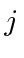axis, the result was.We can think of rotations and boosts as the two basic symmetry transformations that we can make in 4 dimensions. We wish to find the transformation matrices for the equations.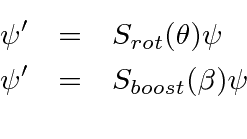We will work with the Dirac equation and its transformation. We know how the Lorentz vectors transform so we can derive a requirement on the spinor transformation. (Remember thatworks in an entirely different space than doand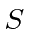.)The transformed equation will be the same as the Dirac equation if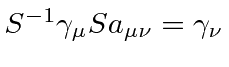. Multiply by the inverse Lorentz transformation.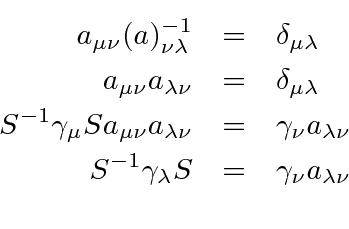This is the requirement onfor covariance of the Dirac equation.

Rotations and boosts are symmetry transformations of the coordinates in 4 dimensions. Consider the cases of rotations about the z axis and boosts along the x direction, as examples.The boost is just another rotation in Minkowski space through and angle. For example a boost with velocity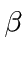in the x direction is like a rotation in the 1-4 plane by an angle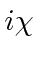. Let us review the Lorentz transformation for boosts in terms of hyperbolic functions. We define.We verify that a boost along thedirection is like a rotation in theplane through an angle.

We need to find the transformation matricesthat satisfy the equation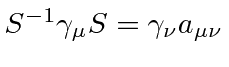for the Dirac equation to be covariant. Recalling that the 4 component equivalent ofis, we will show that these matrices are (for a rotation in the xy plane and a boost in the x direction).Note that this is essentially the transformation that we derived for rotations of spin one-half states extended to 4 components. For the case of the boost the angle is now.

Lets verify that this choice works for a boost.The equation we must satisfy can be checked for eachmatrix. First check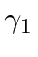. The operations with thematrices all come from the anticommutator,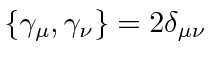, which tells us that the square of any gamma matrix is one and that commuting a pair of (unequal) matrices changes the sign.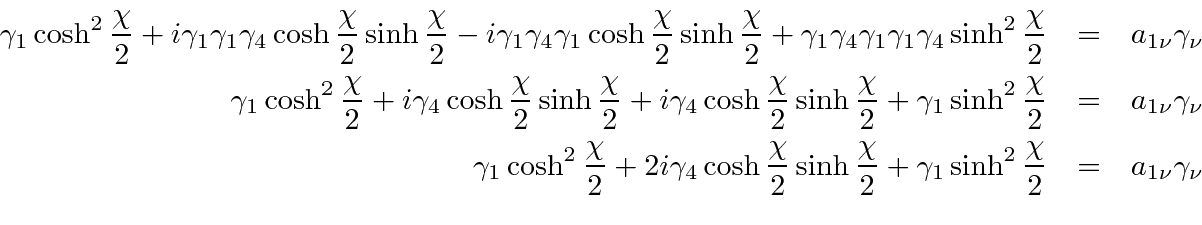That checks for. Now, try.That one also checks. As a last test, try.The Dirac equation is therefore shown to be invariant under boosts along thedirection if we transform the Dirac spinor according to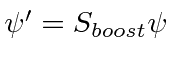with the matrixand.

The pure rotation about the z axis should also be verified.Foror 4,and the requirement is fairly obviously satisfied. Checking the requirement for, we get.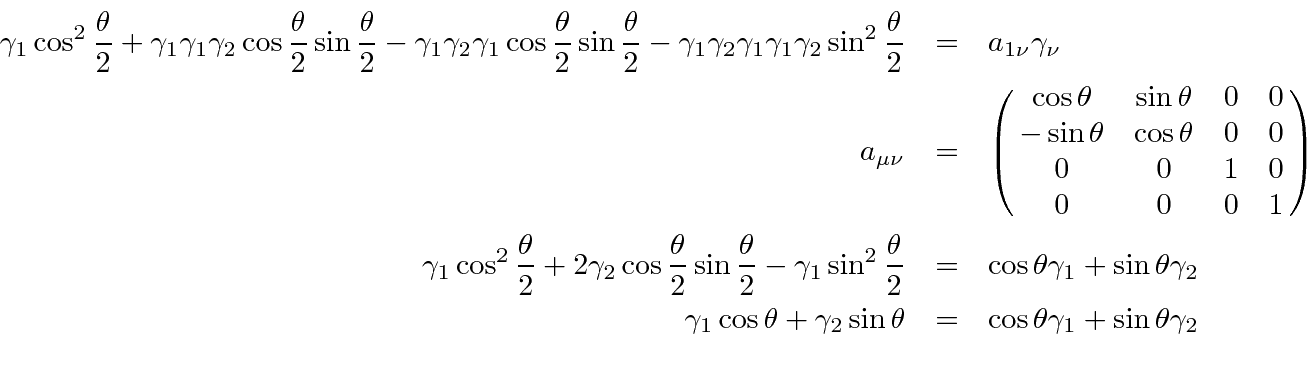This also proves to be the right transformation ofso that the Dirac equation is invariant under rotations about the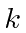axis if we transform the Dirac spinor according towith the matrix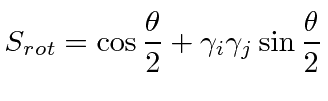andis a cyclic permutation.

Despite the fact that we are using a vector of constant matrices,, the Dirac equation is covariant if we choose the right transformation of the spinors. This allows us to move from one coordinate system to another.

As an example, we might try our solution for a free electron with spin up along the z axis at rest.The solution we found for a free particle with momentumwas.Imagine we boost the coordinate system along thedirection with. We can transform the momentum of the electron to the new frame.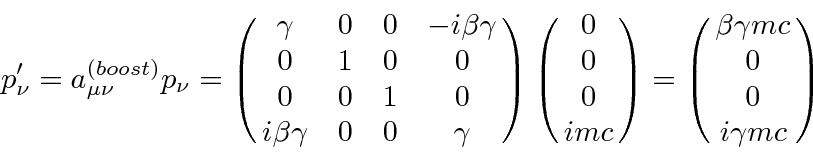The momentum along the x direction is. We now have two ways to get the free particle state with momentum in the x direction. We can use our free particle state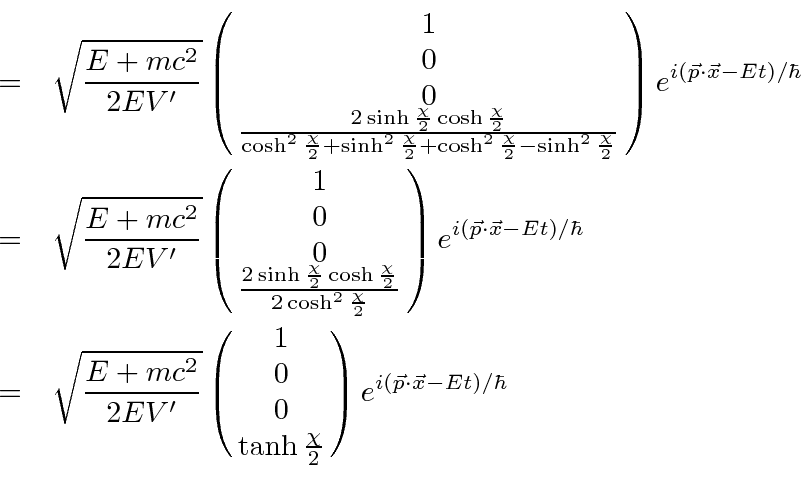where the normalization factor is now set to be, defining this as the primed system.

We can also find the same state by boosting the at rest solution. Recall that we are boosting in the x direction with, implying.In the last step the simple Lorentz contraction was used to set. This boosted state matches the plane wave solution including the normalization.

Jim Branson 2013-04-22